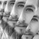# Volume Weighted Average Range Bands [DW]

Dilihat 2368
2368
This is an experimental study designed to identify the underlying trend bias and volatility of an instrument over any custom interval TradingView supports.
First, reset points are established at points where the opening price of the interval changes.
Next, Volume Weighted Average Price ( VWAP ) is calculated. It is the cumulative sum of typical price times volume divided by the cumulative volume . The cumulation starts over upon each reset point.
After that, Volume Weighted Average Range (VWAR) is calculated. The formula structure is the same as VWAP , except using range rather than typical price.
Lastly, the bands are calculated by multiplying the VWAR by the specified multiplier (approximate Golden Ratio by default) and by 1 through 5, then adding to and subtracting from the VWAP .

Custom Bar Colors are included.Efkairia
@Efkairia, The orange bar is not a gap alert. It simply shows that the current bar doesn't meet the bullish or bearish conditions. It is a "neutral" bar
Jawab